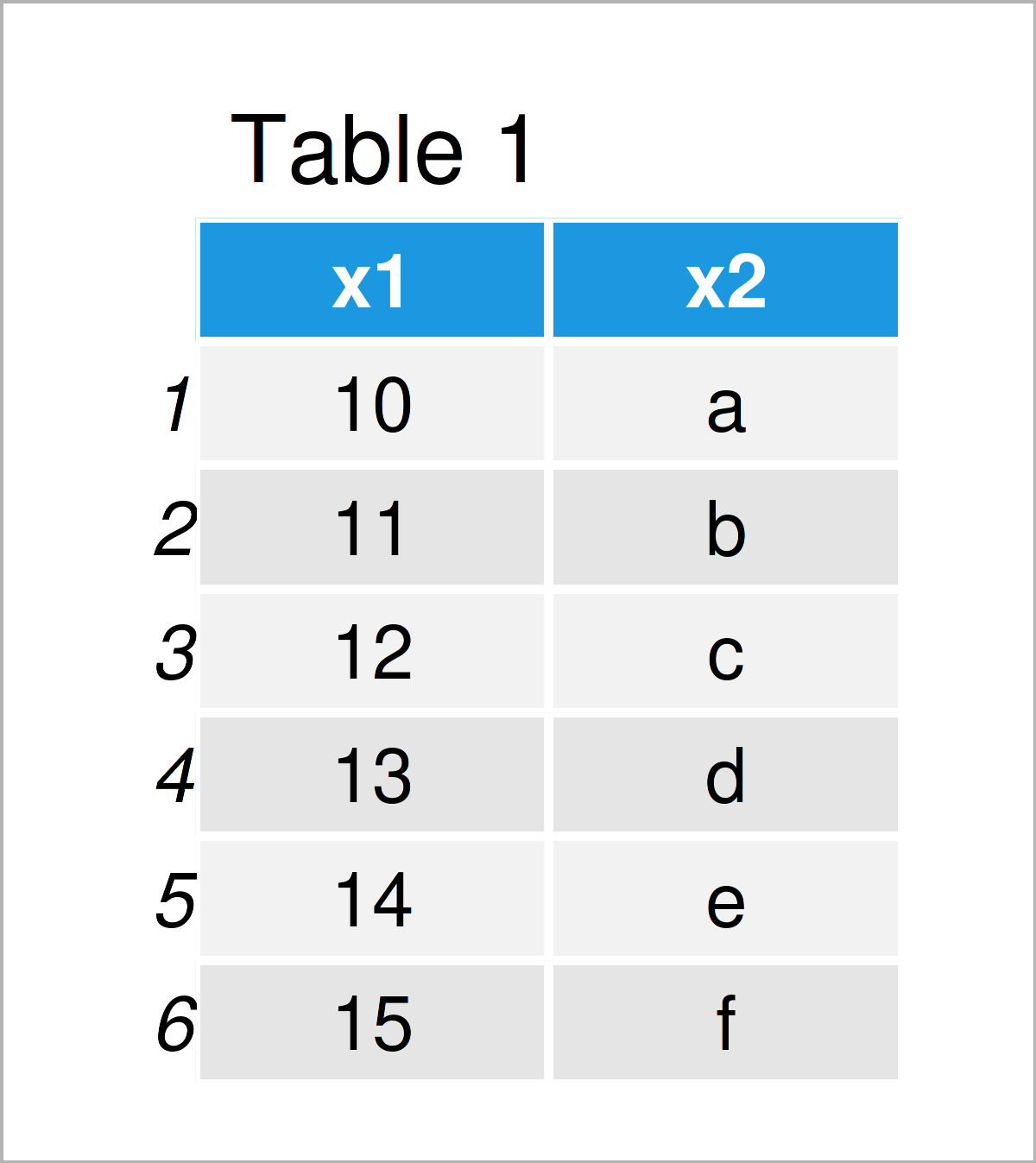# comment Function in R (2 Examples)

This tutorial illustrates how to query or set a comment attribute using the comment() function in R programming.

The tutorial will consist of this:

It’s time to dive into the examples:

## Introduction of Exemplifying Data

Initially, we’ll need to construct some example data:

```data <- data.frame(x1 = 10:15,              # Create example data frame
x2 = letters[1:6])
data                                        # Print example data frame```Table 1 shows that our exemplifying data is made up of six rows and two columns.

## Example 1: Query Comment Attribute Using comment() Function

The following R code explains how to return all comments that have been assigned as attributes to a data object in R.

For this task, we can apply the comment function as shown below:

```comment(data)                               # Check for comments in data frame
# NULL```

The RStudio console returns NULL, i.e. at this point no comments have been attributed to our example data frame.

## Example 2: Set Comment Attribute Using comment() Function

This section shows how to specify a particular attribute for a data object in R.

For this, we can apply the comment function as shown in the following R code:

`comment(data) <- "This is my data frame"    # Set comment`

Let’s query the attributes of our data object once again:

```comment(data)                               # Check for comments in data frame
#  "This is my data frame"```

This time, the comment function has returned the sentence “This is my data frame” that we have assigned as an attribute in the previous step.

As you can see, the comment function can be useful when you want to put some notes on a data object for other users of this data set.

## Video & Further Resources

Do you need more info on the content of the present tutorial? Then you may want to watch the following video on my YouTube channel. In the video, I’m explaining the R syntax of this article in RStudio.

Furthermore, you might read the related articles on my website. You can find a selection of tutorials below.

In summary: In this tutorial, I have explained how to return or set a comment attribute for a data object using the comment() function in R programming. In case you have further comments or questions, let me know in the comments. Furthermore, don’t forget to subscribe to my email newsletter to get updates on new tutorials.

Subscribe to the Statistics Globe Newsletter